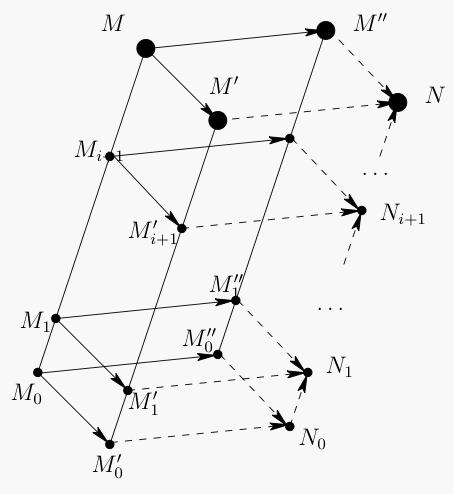Alexei KolesnikovI am interested in Model Theory. In particular, I am studying classification theory questions for first order logic and for non-elementary classes.

A recurring theme in my research is the study of the independence notions and generalized amalgamation properties in various model-theoretic contexts. Generalized amalgamation properties were introduced by Saharon Shelah in 1970s; they were used by him to settle a number of difficult questions. There is good reason to believe that these properties will play a role in providing an answer to Shelah's categoricity conjecture:

If $$\mathcal{K}$$ is an abstract elementary class, then there is a cardinal $$\lambda$$ such that if $$\mathcal{K}$$ is categorical in some cardinal $$\kappa>\lambda$$, then $$\mathcal{K}$$ is categorical in all cardinals above $$\lambda$$.
If $$\mathcal{K}$$ is a class of all models of a complete first-order theory, then it is known that $$\lambda$$ is equal to the cardinality of the language (proved by Morley for countable languages and by Shelah for uncountable languages).

It is also known that for classes that are axiomatized by a sentence of $$L_{\omega_1,\omega}$$ the cardinal $$\lambda$$ has to be at least $$\aleph_\omega$$. This is witnessed by a family of examples due to Hart and Shelah (and generalized amalgamation, as well as its failure, does play a role there). Are there examples showing that $$\lambda$$ has to be larger still? A version of a conjecture states that $$\lambda$$ will be $$\beth_{\omega_1}$$ for a class of models axiomatized by a sentence of $$L_{\omega_1,\omega}$$, a long way up from $$\aleph_\omega$$.

My hope is that by better understanding these properties, we will be able to make progress on the above questions. And a version of the properties formulated for first-order theories offers a convenient testing ground for developing such understanding. The goal here is to obtain as many characterizations of the amalgamation properties as possible.

A recent focus of my research, in collaboration with John Goodrick and Byunghan Kim, had been on describing the amalgamation properties in stable first-order theories in homology-theoretic terms and determining what canonical, in a certain sense, objects witness the failure of the properties.

If you are interested to see the publications that have appeared in print (or have been accepted and will appear in print soon) or to see the list of my recent talks, please follow the links at top of this page.